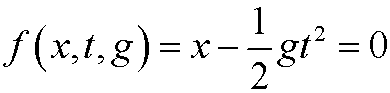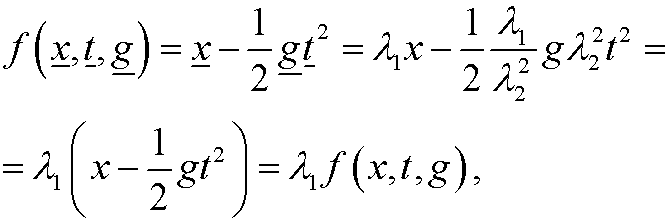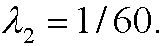4. A unit free physical law.

The physical lawis unit free if it for all positiveholds thatif and only ifwhere qi are definied as in the previous part.

Example:
The physical lawis unit free.

Proof: If we putwe getsinceThe law thus becomesthat is,Remark: If x is given in centimeters and x is given in meters, we have thatIf t is given in seconds and t in minutes, we have thatIn this case, g is given in m/(min)2.